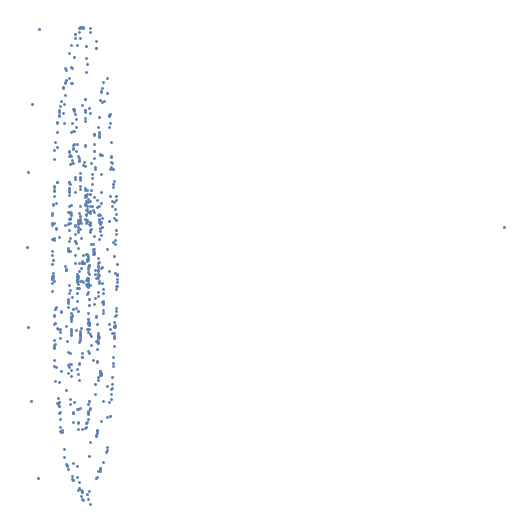#Function Repository Resource:

# WolframModelData

Retrieve information on a Wolfram model from the Registry of Notable Universes

Contributed by: Stephen Wolfram and Bob Sandheinrich
 ResourceFunction["WolframModelData"][code] gives the rule corresponding to the specified short code. ResourceFunction["WolframModelData"][rule] gives the Registry short code corresponding to the specified Wolfram model rule. ResourceFunction["WolframModelData"][rule,"ShortCode"] gives the short code for a published rule. ResourceFunction["WolframModelData"][code,prop] gives the specified property or list of properties associated with a Registry short code. ResourceFunction["WolframModelData"][{code1,code2,…},…] gives data for each codei in a list.

## Details and Options

ResourceFunction["WolframModelData"][] is equivalent to ResourceFunction["WolframModelData"][All] and gives short codes for all published models.
Possible properties include:
 "ConnectedInput" whether the left-hand side of the rule is a connected hypergraph "ConnectedOutput" whether the right-hand side of the rule is a connected hypergraph "ConnectedInputOutputUnion" whether the left-hand side of the rule is connected to the right-hand side "DocumentationLink" the link to the Registry of Notable Universe Models "InitialCondition" the initial state for the model "MaximumArity" the largest number of vertices in any single edge "ReleaseDate" the date the model was published in the Registry "Rule" the hypergraph replacement rule for the model "RuleComplexity" a numerical value correlated with the complexity of the rule "RuleNodeCounts" counts of distinct vertices on each side of the rule "RuleNodesDroppedAdded" counts of vertices only appearing on the left- and right-hand sides of the rule "RuleSignature" counts of edge arities on each side of the rule "RuleSignatureList" unformatted rule signature "ShortCode" the short code in the Registry of Notable Universe Models "TransformationCount" the number of rules in the model "Version" the version of the data format
ResourceFunction["WolframModelData"][code,"Properties"] gives a list of available properties.
ResourceFunction["WolframModelData"][code,All] gives an Association containing all available information.

## Examples

### Basic Examples

Get the rule for a code:

 In:=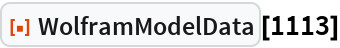Out=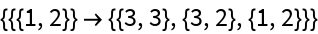Get the full metadata:

 In:=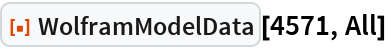Out=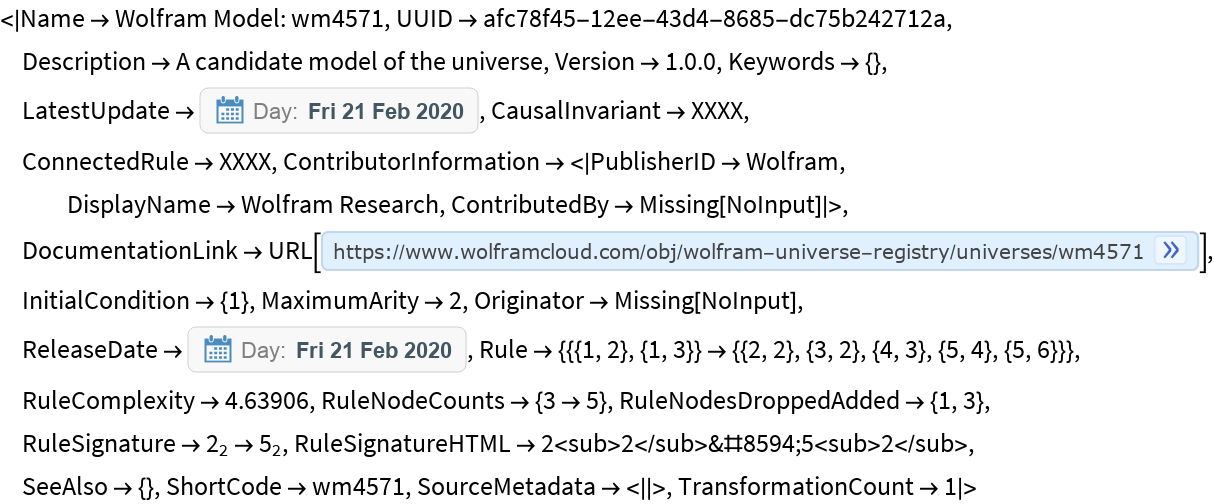Get the code for a published rule:

 In:=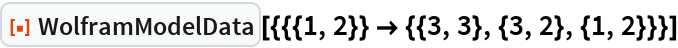Out=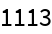### Scope

Codes can also be given as strings:

 In:=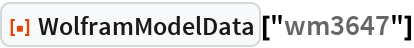Out=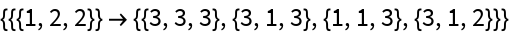Without the "wm" prefix:

 In:=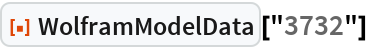Out=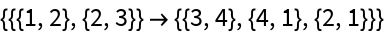Get a specific parameter:

 In:=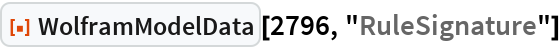Out=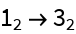Get multiple parameters:

 In:=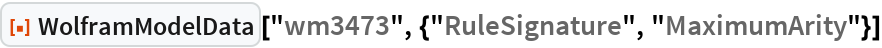Out=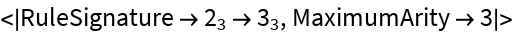See the list of available properties:

 In:=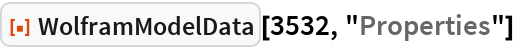Out=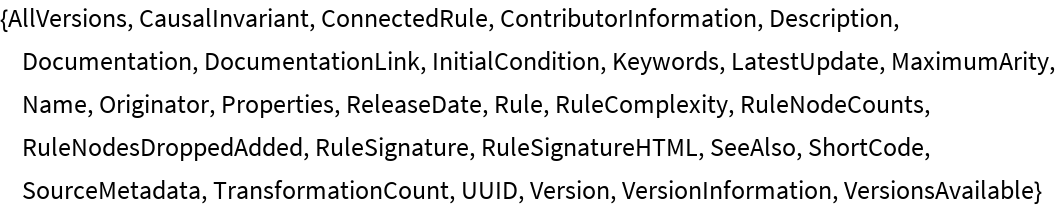Get the rules for a list of models:

 In:=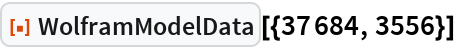Out=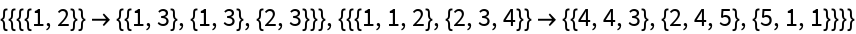Get all the published short codes:

 In:=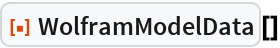Out=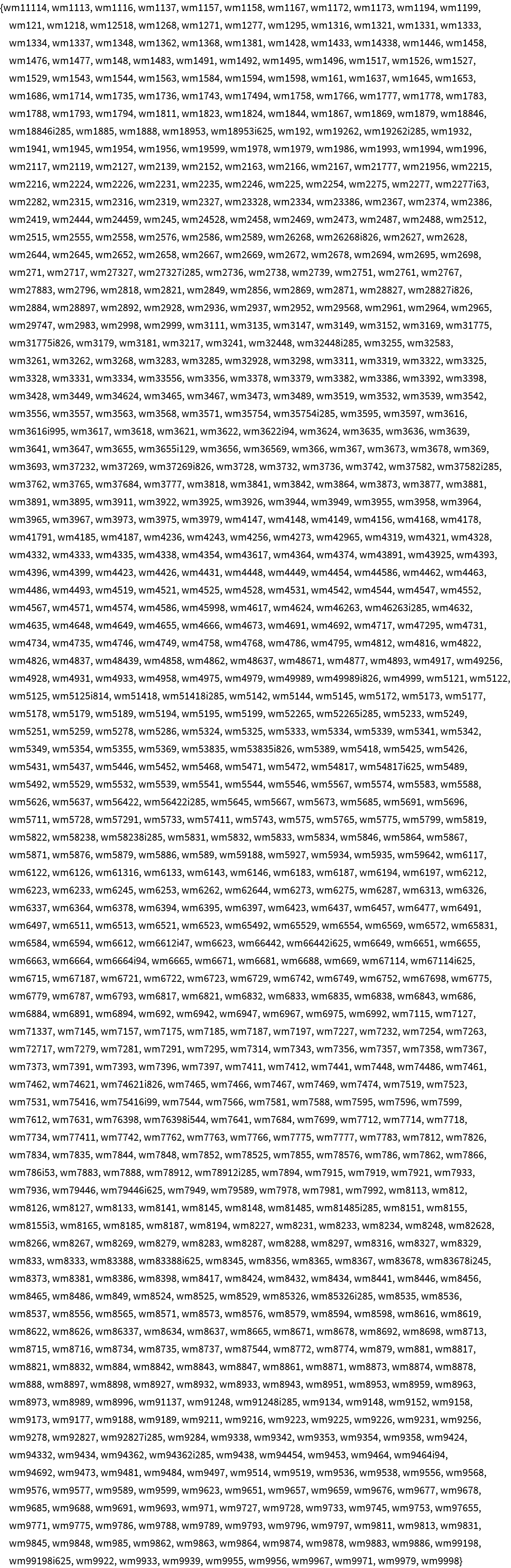Find the most common published rule signatures:

 In:=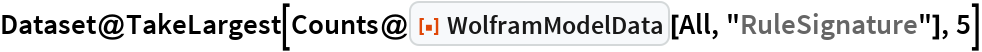Out=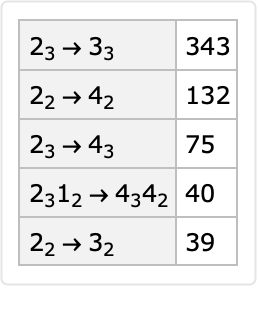Get all the published metadata:

 In:=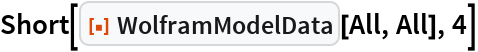Out=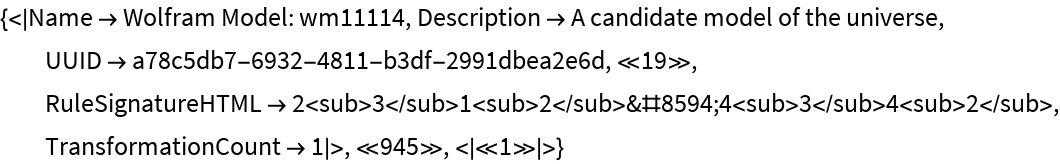Show all the published rules in a feature space:

 In:=Out=